# Properties of solutions

Filed under Basic Science

rate 1 star rate 2 star rate 3 star rate 4 star rate 5 star# Properties of solutions

Michael E. Aulton

Chapter contents

## Introduction

The aim of this chapter is to provide information on certain properties of solutions that relate to their applications in pharmaceutical science. This chapter deals mainly with the physicochemical properties of solutions that are important with respect to pharmaceutical systems. These aspects are covered in sufficient detail to introduce the pharmaceutical scientist to these properties to allow an understanding of their importance in dosage form design and drug delivery. Much is published elsewhere in far greater detail and any reader requiring this additional information can trace some of this by referring to the bibliography at the end of the chapter.

## Types of solution

Solutions may be classified based on the physical state (i.e. gas, liquid or solid) of the solute(s) and solvent. Although a variety of different types can exist, solutions of pharmaceutical interest virtually all possess liquid solvents. In addition, the solutes are predominantly solid substances. Consequently, most of the information in this chapter is relevant to solutions of solids in liquids.

### Vapour pressures of solids, liquids and solutions

An understanding of many of the properties of solutions requires an appreciation of the concept of an ideal solution and its use as a reference system, to which the behaviours of real (non-ideal) solutions can be compared. This concept is itself based on a consideration of vapour pressure. The present section serves as an introduction to the later discussions on ideal and non-ideal solutions.

The kinetic theory of matter indicates that the thermal motion of molecules of a substance in its gaseous state is more than adequate to overcome the attractive forces that exist between the molecules. Thus, the molecules will undergo a completely random movement within the confines of the container. The situation is reversed, however, when the temperature is lowered sufficiently so that a condensed phase is formed. Here the thermal motions of the molecules are now insufficient to overcome completely the intermolecular attractive forces and some degree of order in the relative arrangement of molecules occurs. This condensed state may be either liquid or solid.

If the intermolecular forces are so strong that a high degree of order is brought about, when the structure is hardly influenced by thermal motions, then the substance is usually in the solid state.

In the liquid condensed state, the relative influences of thermal motion and intermolecular attractive forces are intermediate between those in the gaseous and solid states. Thus, the effects of interactions between the permanent and induced dipoles, i.e. the so-called van der Waals forces of attraction, lead to some degree of coherence between the molecules of liquids. Consequently, liquids occupy a definite volume with a surface, unlike gases, and whilst there is evidence of structure within liquids, such structure is much less apparent than in solids.

Although both solids and liquids are condensed systems with cohering molecules, some of the surface molecules in these systems will occasionally acquire sufficient energy to overcome the attractive forces exerted by adjacent molecules. The molecules can therefore escape from the surface to form a vapour phase. If temperature is maintained constant, equilibrium will be established eventually between the vapour phase and the condensed phase. The pressure exerted by the vapour at this equilibrium is referred to as the vapour pressure of the substance.

All condensed systems have the inherent ability to give rise to a vapour pressure. However, the vapour pressures exerted by solids are usually much lower than those exerted by liquids, because the intermolecular forces in solids are stronger than those in liquids. Thus, the escaping tendency for surface molecules is higher in liquids. Consequently, surface loss of vapour from liquids by the process of evaporation is more common than surface loss of vapour from solids by sublimation.

In the case of a liquid solvent containing a dissolved solute, molecules of both solvent and solute may show a tendency to escape from the surface and so contribute to the vapour pressure. The relative tendencies to escape will depend on the relative numbers of the different molecules in the surface of the solution, and on the relative strengths of the attractive forces between adjacent solvent molecules on the one hand and between solute and solvent molecules on the other hand. Because the intermolecular forces between solid solutes and liquid solvents tend to be relatively strong, such solute molecules do not generally escape from the surface of a solution nor contribute to the vapour pressure. In other words, the solute is generally non-volatile and the vapour pressure arises solely from the dynamic equilibrium that is set up between the rates of evaporation and condensation of solvent molecules contained in the solution. In a mixture of miscible liquids, i.e. a liquid in liquid solution, the molecules of both components are likely to evaporate and contribute to the overall vapour pressure exerted by the solution.

### Ideal solutions: Raoult’s Law

The concept of an ideal solution has been introduced in order to provide a model system that can be used as a standard to which real or non-ideal solutions can be compared. In the model, it is assumed that the strengths of all intermolecular forces are identical. Thus solvent/solvent, solute/solvent and solute/solute interactions are the same and are equal to the strength of the intermolecular interactions in either the pure solvent or pure solute. Because of this equality, the relative tendencies of solute and solvent molecules to escape from the surface of the solution will be determined only by their relative numbers in the surface.

Since a solution is homogeneous by definition, then the relative number of these surface molecules will be the same as the relative number in the whole of the solution. The latter can be expressed conveniently by the mole fractions of the components because, for a binary solution (i.e. one with two components), x1 + x2 = 1, where x1 and x2 are the mole fractions of the solute and solvent, respectively.

The total vapour pressure (P) exerted by a binary solution is given by Equation 3.1: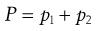(3.1)

where p1 and p2 are the partial vapour pressures exerted above the solution by the solute and solvent, respectively. Raoult’s Law states that the partial vapour pressure (p) exerted by a volatile component in a solution at a given temperature is equal to the vapour pressure of the pure component at the same temperature (po) multiplied by its mole fraction in the solution (x), i.e.: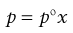(3.2)

Thus from Equations 3.1 and 3.2: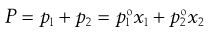(3.3)

where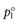and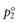are the vapour pressures exerted by pure solute and pure solvent, respectively. If the total vapour pressure of the solution is described by Equation 3.3, then Raoult’s Law is obeyed by the system.

One of the consequences of the preceding comments is that an ideal solution may be defined as one that obeys Raoult’s Law. In addition, ideal behaviour should be expected to be exhibited only by real systems composed of chemically similar components, because it is only in such systems that the condition of equal intermolecular forces between components (as assumed in the ideal model) is likely to be satisfied. Consequently, Raoult’s Law is obeyed over an appreciable concentration range by relatively few systems in reality.

Mixtures of, for example, benzene + toluene, n-hexane + n-heptane, ethyl bromide + ethyl iodide and binary mixtures of fluorinated hydrocarbons are systems that exhibit ideal behaviour. Note the chemical similarity of the two components of the mix in each example.

### Real or non-ideal solutions

The majority of real solutions do not exhibit ideal behaviour because solute–solute, solute–solvent and solvent–solvent forces of interaction are unequal. These inequalities alter the effective concentration of each component so that it cannot be represented by a normal expression of concentration, such as the mole fraction term x that is used in Equations 3.2 and 3.3. Consequently, deviations from Raoult’s Law are often exhibited by real solutions and the previous equations are not obeyed in such cases. These equations can be modified, however, by substituting each concentration term (x) by a measure of the effective concentration; this is provided by the so-called activity (or thermodynamic activity), a. Thus, Equation 3.2 is converted into Equation 3.4: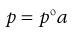(3.4)

and the resulting equation is applicable to all systems whether they are ideal or non-ideal. It should be noted that if a solution exhibits ideal behaviour then a equals x, whereas a will not equal x if deviations from such behaviour are apparent. The ratio of activity divided by the mole fraction is termed the activity coefficient (f) and it provides a measure of the deviation from the ideal. Thus when a = x, f = 1.

If the attractive forces between solute and solvent molecules are weaker than those between the solute molecules themselves or between the solvent molecules themselves, then the components will have little affinity for each other. The escaping tendency of the surface molecules in such a system is increased when compared with an ideal solution. In other words, p1, p2 and therefore P are greater than expected from Raoult’s Law and the thermodynamic activities of the components are greater than their mole fractions, i.e. a1 > x1 and a2 > x2. This type of system is said to show a positive deviation from Raoult’s Law and the extent of the deviation increases as the miscibility of the components decreases. For example, a mixture of alcohol and benzene shows a smaller deviation than the less miscible mixture of water + diethyl ether whilst the virtually immiscible mixture of benzene + water exhibits a very large positive deviation.

Conversely, if the solute and solvent molecules have a strong mutual affinity (that sometimes may result in the formation of a complex or compound), then a negative deviation from Raoult’s Law occurs. Thus, p1, p2 and therefore P are lower than expected and a1 < x1 and a2 < x2. Examples of systems that show this type of behaviour include chloroform + acetone, pyridine + acetic acid and water + nitric acid.

Although most systems are non-ideal and deviate either positively or negatively from Raoult’s Law, such deviations are small when a solution is dilute. This is because the effect that a small amount of solute has on interactions between solvent molecules is minimal. Thus, dilute solutions tend to exhibit ideal behaviour and the activities of their components approximate to their mole fractions, i.e. a1 approximately equals x1 and a2 approximately equals x2. Conversely, large deviations may be observed when the concentration of a solution is high.

Knowledge of the consequences of such marked deviations is particularly important in relation to the distillation of liquid mixtures. For example, the complete separation of the components of a mixture by fractional distillation may not be achievable if large positive or negative deviations from Raoult’s Law give rise to the formation of so-called azeotropic mixtures with minimum and maximum boiling points, respectively.